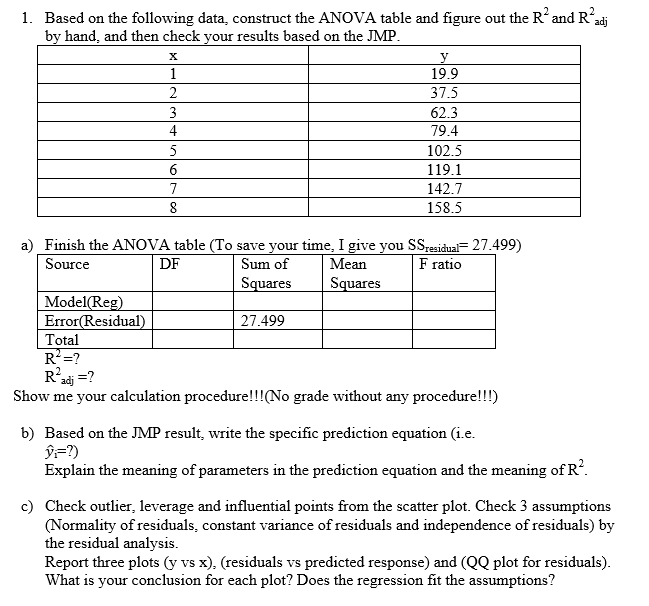### Create an Account

Home / Questions / Based on the following data construct the ANOVA tBased on the following data construct the...

# Based on the following data construct the ANOVA tBased on the following data construct the ANOVA table and figure out the R^2 and R^2_adj by handBased on the following data, construct the ANOVA table and figure out the R^2 and R^2_adj by hand, and then check your results based on the JMP. Finish the ANOVA table (To save your time, I give you SS_residual = 27.499) R^2 =? R^2 _adj =? Show me your calculation procedure!! !(No grade without any procedure!!!) Based on the JMP result, write the specific prediction equation (i.e. y_i =?) Explain the meaning of parameters in the prediction equation and the meaning of R^2. Check outlier, leverage and influential points from the scatter plot. Check 3 assumptions (Normality of residuals, constant variance of residuals and independence of residuals) by the residual analysis. Report three plots (y vs x), (residuals vs predicted response) and (QQ plot for residuals). What is your conclusion for each plot? Does the regression fit the assumptions?

Apr 25 2020 View more View LessSubscribe To Get Solution